# Considering binary numbers and entering correct numbers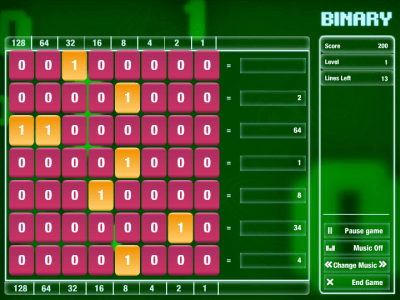A game that converts decimal numbers and binary numbers interchangeably as if it became a resident of the computer world represented by binary numbers of 0 and 1. Since digits for decimal numbers are also written for each digit, it is relatively easy to calculate.

The game is from the following.BINARY game
http://forums.cisco.com/CertCom/game/binary_game.swf

Click START to start.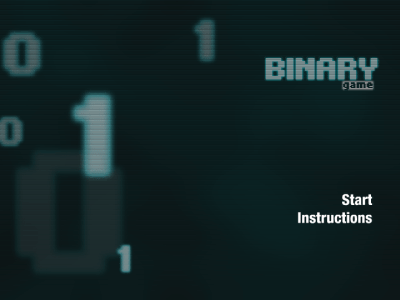The left of the equal sign is a binary number and the right is a decimal number. If there is a number in the square on the right, click on the block on the left to display 1 or 0 and represent the decimal number as a binary number. It is easy to understand since the number in the case of decimal number is written under the block.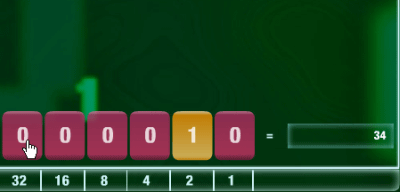If there is no number in the square on the right, represent the binary number in decimal.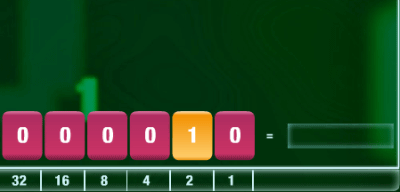Click the square, the numeric keypad will appear so click on the correct number. When you finish entering the number press the Enter key in the lower right. This input can also be done from the keyboard.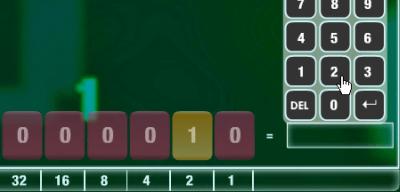Every time over time problems are being added downwards, game over if you go beyond the top frame.It will start over from the beginning.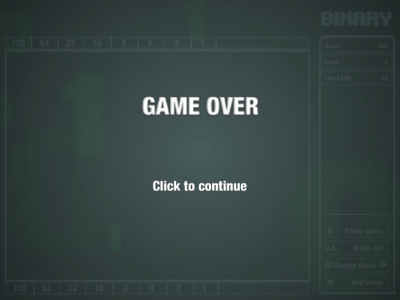in Review,   Game, Posted by darkhorse_log